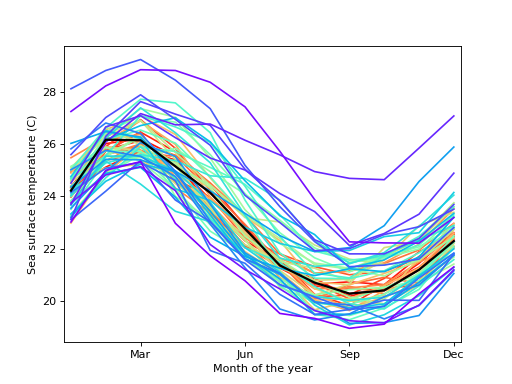# statsmodels.graphics.functional.rainbowplot¶

statsmodels.graphics.functional.rainbowplot(data, xdata=None, depth=None, method='MBD', ax=None, cmap=None)[source]

Create a rainbow plot for a set of curves.

A rainbow plot contains line plots of all curves in the dataset, colored in order of functional depth. The median curve is shown in black.

Parameters
• data (sequence of ndarrays or 2-D ndarray) – The vectors of functions to create a functional boxplot from. If a sequence of 1-D arrays, these should all be the same size. The first axis is the function index, the second axis the one along which the function is defined. So data[0, :] is the first functional curve.

• xdata (ndarray, optional) – The independent variable for the data. If not given, it is assumed to be an array of integers 0..N-1 with N the length of the vectors in data.

• depth (ndarray, optional) – A 1-D array of band depths for data, or equivalent order statistic. If not given, it will be calculated through banddepth.

• method ({'MBD', 'BD2'}, optional) – The method to use to calculate the band depth. Default is ‘MBD’.

• ax (Matplotlib AxesSubplot instance, optional) – If given, this subplot is used to plot in instead of a new figure being created.

• cmap (Matplotlib LinearSegmentedColormap instance, optional) – The colormap used to color curves with. Default is a rainbow colormap, with red used for the most central and purple for the least central curves.

Returns

fig – If ax is None, the created figure. Otherwise the figure to which ax is connected.

Return type

Matplotlib figure instance

See also

References

 R.J. Hyndman and H.L. Shang, “Rainbow Plots, Bagplots, and Boxplots for

Functional Data”, vol. 19, pp. 29-25, 2010.

Examples

Load the El Nino dataset. Consists of 60 years worth of Pacific Ocean sea surface temperature data.

>>> import matplotlib.pyplot as plt
>>> import statsmodels.api as sm
>>> data = sm.datasets.elnino.load(as_pandas=False)


Create a rainbow plot:

>>> fig = plt.figure()
>>> ax = fig.add_subplot(111)
>>> res = sm.graphics.rainbowplot(data.raw_data[:, 1:], ax=ax)

>>> ax.set_xlabel("Month of the year")
>>> ax.set_ylabel("Sea surface temperature (C)")
>>> ax.set_xticks(np.arange(13, step=3) - 1)
>>> ax.set_xticklabels(["", "Mar", "Jun", "Sep", "Dec"])
>>> ax.set_xlim([-0.2, 11.2])
>>> plt.show()